# What does Volts, Amps, Ohms, and Watts mean?

Standard units of measurement are established by the official organization that is tasked with the standardization of international weights and measurements, ensuring that the entire world uses the same standards of weight and measurement. The French organization is called Bureau International des Poids et Mesures, or BIPM, translated to English as International Bureau of Weights and Measures. The definitions on this page are adopted from the official definitions which can be found in BIPM's International System of Units, or SI. References and links are included for each defined term which refer to information provided by BIPM.

## What is a volt?

A "volt" is a unit of electric potential, also known as electromotive force, and represents "the potential difference between two points of a conducting wire carrying a constant current of 1 ampere, when the power dissipated between these points is equal to 1 watt." Stated another way, a potential of one volt appears across a resistance of one ohm when a current of one ampere flows through that resistance.  Volts can be expressed in to SI base units thusly: 1 V = 1 kg times m2 times s-3 times A-1 (kilogram meter squared per second cubed per ampere), or...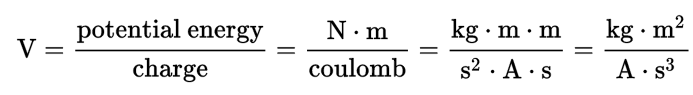#### What is voltage?

"Voltage" (V) is the potential for energy to move and is analogous to water pressure.  The characteristics of voltage are like that of water flowing through pipes.  This is known as the "water-flow analogy", which is sometimes used to explain electric circuits by comparing them with a closed system of water-filled pipes, or "water circuit", that is pressurized by a pump.  Refer to the image below to visualize how voltage and electric current works...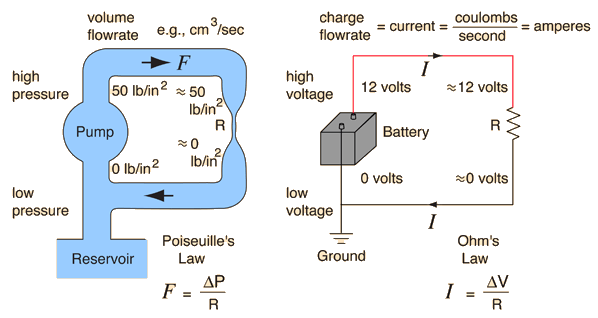Current (I) is a rate of flow and is measured in amps (A). Ohms (R) is a measure of resistance and is analogous to the water pipe size.  Current is proportional to the diameter of the pipe or the amount of water flowing at that pressure.

Voltage is an expression of the available energy per unit charge which drives the electric current around a closed circuit in a direct current (DC) electrical circuit. Increasing the resistance, comparable to decreasing the pipe size in the water circuit, will proportionately decrease the current, or water flow in the water circuit, which is driven through the circuit by the voltage, which is comparable to the hydraulic pressure in a water circuit.

The relationship between voltage and current is defined (in ohmic devices like resistors) by Ohm's Law. Ohm's Law is analogous to the Hagen–Poiseuille equation, as both are linear models relating flux and potential in their respective systems.  Electric current (I) is a rate of flow and is measured in amps (A). Ohms (R) is a measure of resistance and is comparable to the water pipe size.## What is an amp?

An "amp", short for ampere, is a unit of electrical current which SI defines in terms of other base units by measuring the electromagnetic force between electrical conductors carrying electric current.  The ampere is that constant current which, if maintained in two straight parallel conductors of infinite length, of negligible circular cross-section, and placed one metre apart in vacuum, would produce between these conductors a force equal to 2×10−7 newtons per metre of length.

#### What is amperage?

"Amperage" is the strength of a current of electricity expressed in amperes.

## What is an ohm?

"Ohm" is a unit of an electric circuit that is defined as the electrical resistance between two points of a conductor when a constant potential difference of one volt, applied to these points, produces in the conductor a current of one ampere, the conductor not being the seat of any electromotive  force.[3An ohm is expressed as...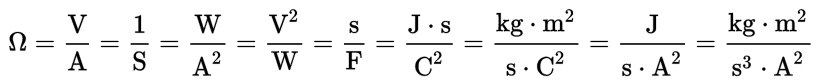## What is a watt?

A "watt" is a measure of power.  One watt (W) is the rate at which work is done when one ampere (A) of current flows through an electrical potential difference of one volt (V).  A watt can be expressed as...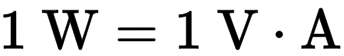## How do all of these terms relate to solar power?

It is important to know the terms and formulas on this page because they are helpful in calculating the amount of power and the size of a solar power system, whether it is an off-grid system or one that is grid-connected.

There is also a formula for power.  In this formula, P is power, measured in watts, I is the current, measured in amperes, and V is the potential difference (or voltage drop) across the component, measured in volts.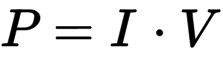A lot of times this is also displayed as W = V * A or watts equals volts multiplied by amps.

Lets re-order this formula for an example:

• W = V * A
• V = W / A
• A = W / V

This example will show why a higher DC voltage is best in large solar systems.

Lets say you have 1000 watts of loads to run. This is equal to:

• 83.3 amps at 12 volts
• 41.6 amps at 24 volts
• 20.8 amps at 48 volts
• 8.3 amps at 120 volts
• 4.1 amps at 240 volts

Knowing how much current is flowing to your load is very important in selecting the correct wire. We take the distance into consideration to calculate the voltage loss. Ideally we don't want to exceed a 3% voltage loss. The other half of this calculation is the current. You need a larger wire to move more current. If you have a choice the higher voltage is best.

These formulas are also useful in calculating AC (alternating current) wattage to determine the size of an inverter, which converts the DC electricity from a solar array to AC that can then be used to power lights and appliances in homes and businesses. Appliances include a face plate which contains all of its electrical data. Lets suppose you have a microwave oven.  The manufacturer will list an amp requirement on the electrical data of the face plate, which is usually attached to the back of the oven. Let's say that the rating on the face plate is 8.3 amps.  To calculate the watts, multiply 8.3 amps by the home's voltage of 120 volts. This equals 996 watts.

Now, lets calculate how much power the microwave will use in one day. If you use the microwave for 2 hours a day, then multiply the hours per day by the watts to get watt-hours per day.  So, you have 996 watts multiplied by 2 hours, which equals 1992 watt-hours per day.

When sizing a solar power system, this formula is necessary in determining the total power you use per day.

Watts = Amps x Volts

Volt = Watts / Amps

Amps = Watts / Volts

##### Footnotes
1.  SI Brochure: The International System of Units (SI) [8th edition, 2006; updated in 2014] - Text in English (PDF)
2.  SI Brochure: The International System of Units (SI) [8th edition, 2006; updated in 2014] - Text in English (PDF)
3.  BIPM SI Brochure: Appendix 1, p. 144 (PDF)